Next: The general case: multiple Up: .  Introduction: Variable gain image Previous: Estimation with more than

## Estimation with unknown exposure values

Generally the relative exposure values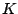are not known a-priori. Thus simultaneously solving for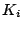and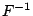is an optimization problem:
• Initially, pairwise comparagrams are constructed and unrolled, each assuming an arbitrary value of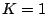(e.g.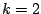). The resulting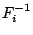function estimates for each pair of images will have the same overall shape, but each be scaled differently, apart from noise.
• Next these estimates ofare used to estimate the relative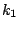values. Without loss of generality,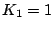may be assumed, and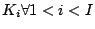may be found.
• Next the estimates ofare consolidated (averaged together) by using the above estimates ofto register them. This registered and averaged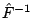is now used to re-estimate thevalues, by comparing ratios: K_i = F^-1 - Q where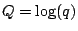is the logarithm of the reference quantity of light. (At this point, this first guess is often good enough to stop, but may undergo successive refinements by continuing as follows...)
• Finally, if desired, images are sorted in increasing order of exposure (least to greatest), and a final estimate ofis made across all pairs of images, using the estimates of.
• If desired, this process may again be repeated, e.g. using that estimateto again estimateand so on.

SubsectionsNext: The general case: multiple Up: .  Introduction: Variable gain image Previous: Estimation with more than
Steve Mann 2002-05-25